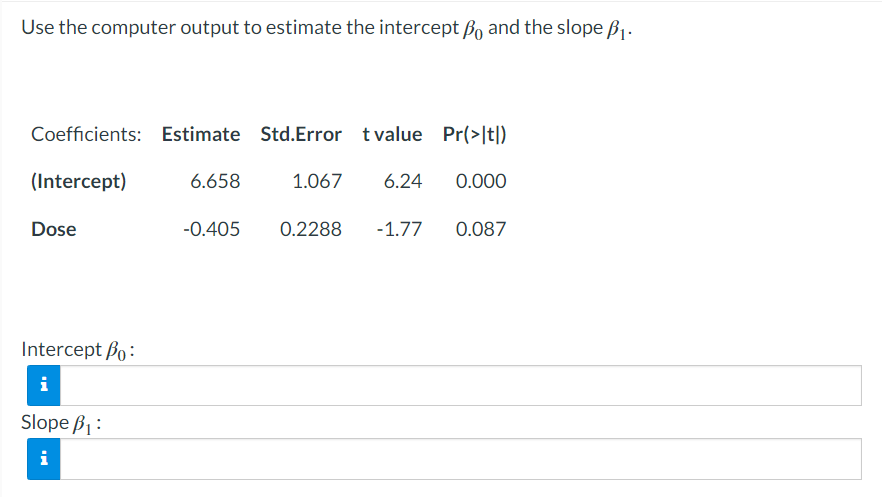Home / Expert Answers / Statistics and Probability / use-the-computer-output-to-estimate-the-intercept-beta-0-and-the-slope-beta-1-i-pa879

# (Solved): Use the computer output to estimate the intercept $$\beta_{0}$$ and the slope $$\beta_{1}$$. I ...Use the computer output to estimate the intercept $$\beta_{0}$$ and the slope $$\beta_{1}$$. Intercept $$\beta_{0}:$$

We have an Answer from Expert